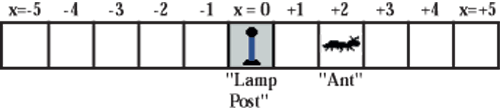HandsOn 11 - Ten-Step Random Walk

It is easiest to visualize random motion (random walk) along one line, that is, in one dimension. Call x the position of the ant (i.e., walker) on a one-dimensional line. Locate the origin, that is x = 0, at the lamp post. Then let each "step'' of the ant-right or left along the line-be of equal length. One way to picture this is to use one row of a checkerboard or a printout of Figure 3.4.Figure 3.4: Diagram of a wandering ant. How many steps do you think he has already taken if his starting-point was at the "Lamp post''?

Choose the direction of the step the ant will take by flipping a penny:

• If it is a head, the ant steps right and x increases by one.

• If it is a tail, the ant steps left and x decreases by one.

A head or tail is equally likely; therefore it is equally probable that the ant steps right or left.

Do this activity with a partner. Use a silver-colored coin (nickel, dime, or quarter) to represent the position of the ant. To begin, put the "ant'' in a center cell (the position of the lamp post). The ant steps from one cell to the next, right or left randomly, depending on whether the penny comes up heads or tails, respectively.

1. Flip a penny ten times and move your "ant'' accordingly.

2. After ten steps, report the final position of the ant, and whether it is to the right or to the left of the lamp post.

3. Again, the tally keeper puts a big X on a bar graph of the final position on the blackboard, as you did in HandsOn 10 (Figure 3.2).

 Q3.14: How does your result compare with the results of HandsOn 10, in which 10 pennies are flipped at once? Is there a relationship between these two activities? Notice that in this present activity the final number of steps is equal to the number of heads minus the number of tails).

Previous: 3.3 - Random Walks

Next: SimuLab 4 - One-Dimensional Random Walk